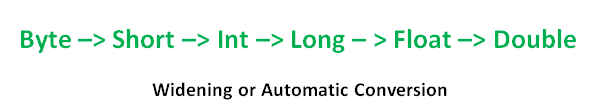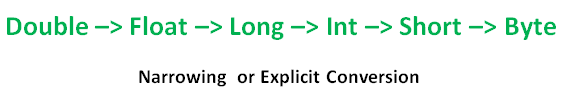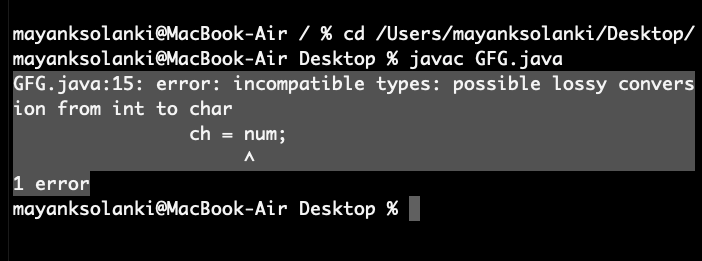# Type conversion in Java with Examples

• Difficulty Level : Easy
• Last Updated : 22 Nov, 2021

Java provides various data types just likely any other dynamic languages such as boolean, char, int, unsigned int, signed int, float, double, long, etc in total providing 7 types where every datatype acquires different space while storing in memory. When you assign a value of one data type to another, the two types might not be compatible with each other. If the data types are compatible, then Java will perform the conversion automatically known as Automatic Type Conversion, and if not then they need to be cast or converted explicitly. For example, assigning an int value to a long variable.

## Widening or Automatic Type Conversion

Widening conversion takes place when two data types are automatically converted. This happens when:

• The two data types are compatible.
• When we assign a value of a smaller data type to a bigger data type.

For Example, in java, the numeric data types are compatible with each other but no automatic conversion is supported from numeric type to char or boolean. Also, char and boolean are not compatible with each other.Example:

## Java

 `// Java Program to Illustrate Automatic Type Conversion` `// Main class``class` `GFG {` `    ``// Main driver method``    ``public` `static` `void` `main(String[] args)``    ``{``        ``int` `i = ``100``;` `        ``// Automatic type conversion``        ``// Integer to long type``        ``long` `l = i;` `        ``// Automatic type conversion``        ``// long to float type``        ``float` `f = l;` `        ``// Print and display commands``        ``System.out.println(``"Int value "` `+ i);``        ``System.out.println(``"Long value "` `+ l);``        ``System.out.println(``"Float value "` `+ f);``    ``}``}`

Output

```Int value 100
Long value 100
Float value 100.0```

## Narrowing or Explicit Conversion

If we want to assign a value of a larger data type to a smaller data type we perform explicit type casting or narrowing.

• This is useful for incompatible data types where automatic conversion cannot be done.
• Here, the target type specifies the desired type to convert the specified value to.char and number are not compatible with each other. Let’s see when we try to convert one into another.

## Java

 `// Java program to illustrate Incompatible data Type``// for Explicit Type Conversion` `// Main class``public` `class` `GFG {` `    ``// Main driver method``    ``public` `static` `void` `main(String[] argv)``    ``{` `        ``// Declaring character variable``        ``char` `ch = ``'c'``;``        ``// Declaringinteger variable``        ``int` `num = ``88``;``        ``// Trying to insert integer to character``        ``ch = num;``    ``}``}`

Output: An error will be generatedThis error is generated as an integer variable takes 4 bytes while character datatype requires 2 bytes. We are trying to plot data from 4 bytes into 2 bytes which is not possible.

How to do Explicit Conversion?

## Java

 `// Java program to Illustrate Explicit Type Conversion` `// Main class``public` `class` `GFG {` `    ``// Main driver method``    ``public` `static` `void` `main(String[] args)``    ``{` `        ``// Double datatype``        ``double` `d = ``100.04``;` `        ``// Explicit type casting by forcefully getting``        ``// data from long datatype to integer type``        ``long` `l = (``long``)d;` `        ``// Explicit type casting``        ``int` `i = (``int``)l;` `        ``// Print statements``        ``System.out.println(``"Double value "` `+ d);` `        ``// While printing we will see that``        ``// fractional part lost``        ``System.out.println(``"Long value "` `+ l);` `        ``// While printing we will see that``        ``// fractional part lost``        ``System.out.println(``"Int value "` `+ i);``    ``}``}`

Output

```Double value 100.04
Long value 100
Int value 100```

Note: While assigning value to byte type the fractional part is lost and is reduced to modulo 256(range of byte).

Example:

## Java

 `// Java Program to Illustrate Conversion of``// Integer and Double to Byte` `// Main class``class` `GFG {` `    ``// Main driver method``    ``public` `static` `void` `main(String args[])``    ``{``        ``// Declaring byte variable``        ``byte` `b;` `        ``// Declaring and initializing integer and double``        ``int` `i = ``257``;``        ``double` `d = ``323.142``;` `        ``// Display message``        ``System.out.println(``"Conversion of int to byte."``);` `        ``// i % 256``        ``b = (``byte``)i;` `        ``// Print commands``        ``System.out.println(``"i = "` `+ i + ``" b = "` `+ b);``        ``System.out.println(``            ``"\nConversion of double to byte."``);` `        ``// d % 256``        ``b = (``byte``)d;` `        ``// Print commands``        ``System.out.println(``"d = "` `+ d + ``" b= "` `+ b);``    ``}``}`

Output

```Conversion of int to byte.
i = 257 b = 1

Conversion of double to byte.
d = 323.142 b= 67```

## Type Promotion in Expressions

While evaluating expressions, the intermediate value may exceed the range of operands and hence the expression value will be promoted. Some conditions for type promotion are:

1. Java automatically promotes each byte, short, or char operand to int when evaluating an expression.
2. If one operand is long, float or double the whole expression is promoted to long, float, or double respectively.

Example:

## Java

 `// Java program to Illustrate Type promotion in Expressions` `// Main class``class` `GFG {` `    ``// Main driver method``    ``public` `static` `void` `main(String args[])``    ``{` `        ``// Declaring and initializing primitive types``        ``byte` `b = ``42``;``        ``char` `c = ``'a'``;``        ``short` `s = ``1024``;``        ``int` `i = ``50000``;``        ``float` `f = ``5``.67f;``        ``double` `d = .``1234``;` `        ``// The Expression``        ``double` `result = (f * b) + (i / c) - (d * s);` `        ``// Printing the result obtained after``        ``// all the promotions are done``        ``System.out.println(``"result = "` `+ result);``    ``}``}`

Output

`result = 626.7784146484375`

## Explicit Type Casting in Expressions

While evaluating expressions, the result is automatically updated to a larger data type of the operand. But if we store that result in any smaller data type it generates a compile-time error, due to which we need to typecast the result.

Example:

## Java

 `// Java program to Illustrate Type Casting``// in Integer to Byte` `// Main class``class` `GFG {` `    ``// Main driver method``    ``public` `static` `void` `main(String args[])``    ``{` `        ``// Declaring byte array``        ``byte` `b = ``50``;` `        ``// Type casting int to byte``        ``b = (``byte``)(b * ``2``);` `        ``// Display value in byte``        ``System.out.println(b);``    ``}``}`

Output

`100`

Note: In case of single operands the result gets converted to int and then it is typecast accordingly, as in the above example.